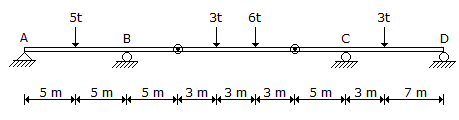# Civil Engineering - Applied Mechanics

### Exercise :: Applied Mechanics - Section 5

31.

The frequency of oscillation on moon as compared to that on earth, will be

 A. 2.44 times more B. 2.44 times less C. 3 times less D. 3 times more.

Explanation:

No answer description available for this question. Let us discuss.

32.

Moment of interia of a squares of side fa about an axis through its centre of gravity, is

 A. b3/4 B. b4/12 C. b4/3 D. b4/8 E. b4/36.

Explanation:

No answer description available for this question. Let us discuss.

33.

The reaction at the support D of the continuous beam ABCD, hinged at two points shown in below figure isA. 1.6 t ↑ B. 1.6 t ↓ C. 0.5 t ↑ D. 0.5 t ↓ E. 8.5 t ↑

Explanation:

No answer description available for this question. Let us discuss.

34.

When a body slides down an inclined surface, the acceleration (f) of the body, is given by

 A. f = g B. f = g sin θ C. f = g cos θ D. f = g tan θ.

Explanation:

No answer description available for this question. Let us discuss.

35.

The velocity ratio of an inclined plane of inclination θ with horizontal for lifting a load is

 A. sin θ B. cos θ C. tan θ D. sec θ E. cosec θ.